StatLect

# Consistent estimator

An estimator of a given parameter is said to be consistent if it converges in probability to the true value of the parameter as the sample size tends to infinity.## The main elements of an estimation problem

Before providing a definition of consistent estimator, let us briefly recall the main elements of a parameter estimation problem:

• a sample of data drawn from an unknown probability distribution; we denote the sample by, where the subscriptis the sample size, that is, the number of observations in the sample;

• a parameter of the unknown data-generating distribution, denoted by(e.g., the mean of a univariate distribution or the correlation coefficient of a bivariate distribution);

• an estimator, which is a function that associates an estimateto each samplethat could possibly be observed.

## Sampling variability

Before being observed, the sampleis regarded as random.

Therefore,, which depends on, is a random variable.

When needed, we write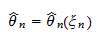to highlight the fact that the estimatoris a function of the sample.

## Definition

Now, imagine that we are able to collect new data and increase our sample sizeindefinitely, so as to obtain a sequence of samplesand a sequence of estimators.

If this "imaginary" sequence of estimators converges in probability to the true parameter value, then it is said to be consistent.

Definition A sequence of estimatorsis said to be consistent if and only if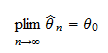wheredenotes convergence in probability.

## Terminology

Note that we have defined "consistent sequences of estimators".

But what do we mean by "consistent estimator"? The latter locution is informally used to mean that:

1. the same predefined rule is used to generate all the estimators in the sequence;

2. the terms of the sequence converge in probability to the true parameter value.

Thus, the concept of consistency extends from the sequence of estimators to the rule used to generate it.

For instance, suppose that the rule is to "compute the sample mean", so thatis a sequence of sample means over samples of increasing size.

Ifconverges in probability to the mean of the distribution that generated the samples, then we say that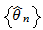is consistent.

By a slight abuse of language, we also say that the sample mean is a consistent estimator.

## Examples

The following table contains examples of consistent estimators (with links to lectures where consistency is proved).

Estimator Estimated parameter Lecture where proof can be found
Sample mean Expected value Estimation of the mean
Sample variance Variance Estimation of the variance
OLS estimator Coefficients of a linear regression Properties of the OLS estimator
Maximum likelihood estimator Any parameter of a distribution Maximum likelihood

## Inconsistent estimator

An estimator which is not consistent is said to be inconsistent.

## Consistent and asymptotically normal

You will often read that a given estimator is not only consistent but also asymptotically normal, that is, its distribution converges to a normal distribution as the sample size increases.

You might think that convergence to a normal distribution is at odds with the fact that consistency implies convergence in probability to a constant (the true parameter value).

In other words, you might ask yourself: "Is convergence to a constant or to a distribution?"

To answer this question, we should give a more precise definition of asymptotic normality.

Consider the ratio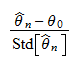Whenis consistent, both the differenceand the standard deviation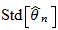converge to zero astends to infinity. However, their ratio can converge to a distribution. When it converges to a standard normal distribution, then the sequenceis said to be asymptotically normal.

The practical consequence of asymptotic normality is that, whenis large, we can approximate the above ratio with a standard normal distribution.

It follows thatcan be approximated by a normal distribution with meanand standard deviation. But the latter converges to zero, so that the distribution becomes more and more concentrated around the mean, ultimately converging to a constant.

## More details

Consistency is discussed in more detail in the lecture on Point estimation.

Previous entry: Conditional probability mass function

Next entry: Convergence criterion